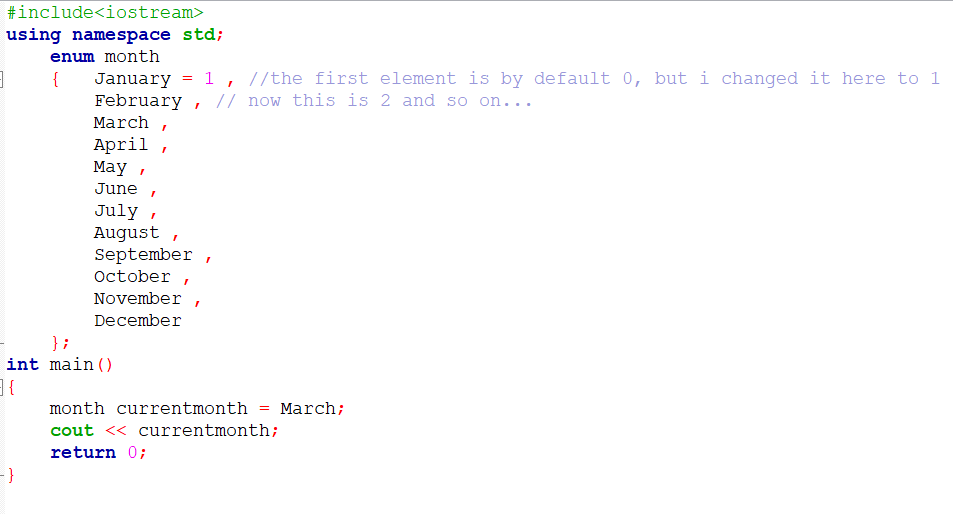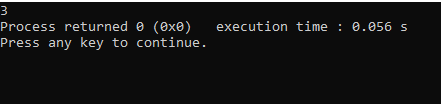# Enumeration in C++

In this tutorial, we are going to learn about the concept of Enumeration, or enum, which is a datatype, and an interesting concept. We will see an example, which demonstrates the concept so that it becomes easy to understand for you, and you will be comfortable using the concept in your C++ programs.

## Enumeration in CPPEnumeration is a kind of user-defined data type, which consists of named values, which represent integral constants. We will understand this with help of an example ahead. In order to define an enumeration, we have to use the “enum” keyword.

### Enum in C++ Example

Have a look at a simple example, through which, we can understand the concept of enumeration-output –So, as you can see that we created a variable of type month, which is an enumeration. By default, the first value in the enum has a value of 0, but here I made it 1 to just show that you can modify those values too… you can try putting 90 to July, and you will see that August will now have a value of 91. If you do not assign anything to January, then it would be zero, and the current month variable which contains the value of March would have a value of 2(instead of 3).

In simple words, we can understand enumeration as a data type, which consists of named values, which represent integral constants, just like in the above example, January represents 1, February represents 2, and so on.

#### Summary

In this tutorial, we learned about enum or enumeration in C++. The concept is very interesting and useful at times when we need to have named values representing integral constants. We saw an example, which demonstrates the concept, and its application.

You can also try some more programs, and some more use cases, for the enumeration.

If you find this tutorial to be interesting, you can explore our other tutorials related to C++ programming language to learn more about C++ programming language. You can also explore our other courses, related to Python, Machine learning, and Data Science, through which, you can upskill yourself.

### Q: What is an enum in C / C++?

Ans: In C / C++, an enum is a datatype, which consists of named values, which represent integral constants.

### Q: How can we create an enum in C++?

Ans: To create an enum in C++, we need to use the keyword enum.

### Q: Is an enum a keyword in C++?

Ans: Yes, “enum” is a keyword in C++, used to declare new enumeration types in programs.